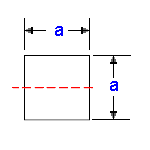#### Area Moment of Inertia Section Properties of Square Calculator

This engineering calculator will determine the Area Moment of Inertia Section Properties and section modulus for the given cross-section. This engineering data is often used in the design of structural beams or structural flexural members. There are two types of section moduli, the elastic section modulus (S) and the plastic section modulus (Z).

See Area Moment of Inertia Section Properties Properties Menu for additional structural section properties equations and calculators.

Area Moment of Inertia Section Properties Square at Center

 Description Equation Area Moment of Inertia Section Properties = ISection Modulus = Z=I/yRadius of GyrationA = areay = distance from axis to extreme fiberArea Moment of Inertia Section Properties: Square Variables Inputs: Inch (in.) Metric (mm) "a"  (Side of Square) = Calculations: Area Moment of Inertia Section Properties (Units4) I = Section Modulus (Units3) Z = Radius of Gyration (Units) = Extreme Point (Units) y = Area (Units2) A =